Monday , May 23 2022# Electricity: 10th CBSE Science Physics

2. #### The element of heating electrical appliances are made-up of an alloy rather than pure metal.

1. (i) High resistivity. (ii) High melting point.
2. (i) High resistivity, high melting point.
(ii) Do not oxidize or burn at high temperature.

2. #### What happens to the reading of A1, A2, A3 and a when the bulb B2 gets fused?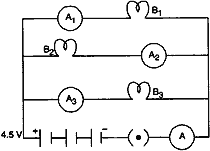1. The glow of the bulbs B2 and B3 will remain the same.
2. A2 shows 1 ampere, A2 shows zero, A3 shows 1 ampere and A shows 2 ampere.

#### Question: A child has drawn the electric to study Ohm’s law as shown in figure. His teacher told that the circuit diagram needs correction. Study the circuit diagram and redraw it after making all corrections.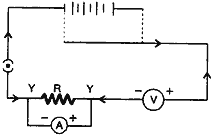Answer: The correct diagram is as shown: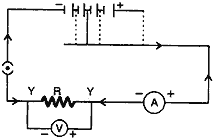#### Question: Why do the wires connecting an electric heater to the mains not glow while its heating element does?

Answer: The wire of the connecting cord of electric heater are made of its extremely low resistance. The heating element of an electric heater made of nichrome wire. It glows because large amount of heat is produced due to its high resistance.

#### Question: In the circuit diagram shown, the two resistance wires A and B are of same area of cross-section and same material, but A is longer than B. Which ammeter A1 or A2 will indicate higher reading for current? Give reason.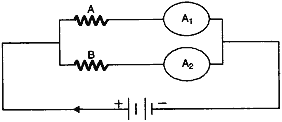Answer: Ammeter A2 shows higher reading. Because, since wire A is longer; it has greater resistance and so draw lesser current. So more current flows through B and A2 shows more reading.

#### Question: How would the reading of voltmeter (V) change, if it is connected between B and C? Justify your answer.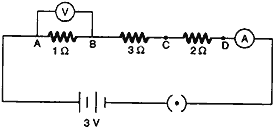R = R1 + R2 + R3 = 1+2+3 = 6 Ω
V = IR
I = V/R = 3/6 = 1/2 A

Voltage across 1 Ω, V = IR = 1/2 × 1 = 0.5 V

Voltage across 3 Ω, V = IR = 1/2 × 3 = 1.5 V

#### Question: In the circuit diagram shown, the two resistance wire A and B are of same length and same material, but A is thicker than B. Which ammeter A1 or A2 will indicate higher reading for current? Give reason.Answer: Ammeter A1 will show higher reading. Because, as wire A is thicker than B, A has lesser resistance. So current drawn by Afrom the battery will be more and hence ammeter A1 will show higher reading.

#### Question: Tungsten is used almost exclusively for filaments of electric bulb. List two reasons.

Answer: Resistivity of tungsten is very high as it is an alloy. It does not oxidise readily at very high temperature.

#### Question: Three V-I graphs are drawn individually for two resistors and their series combination. Out of A, B, C which one represents the graph for series combination of the other two. Give reason for your answer.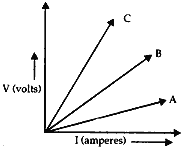Answer: We know that in series combination, resultant resistance in always greater than the individual resistances. In the given graph, C is shown maximum resistance (as slope of V-I graph gives resistance), thus, C represents the resultant series combination of the other two.

#### Question: Mention the condition under which charges can move in a conductor. Name the device which is used to maintain this condition in a electric circuit.

Answer: Charges can move, if there is a potential difference across the two ends of the conductor. Electric cell or a battery consisting of one or more cells can maintain electric current in an electric circuit.

#### Question: (1). Name the effect of electric current which is utilized in the working of an electrical fuse? (2). How is a fuse connected in a domestic circuit?

1. Heating effect.
2. Always in series with live wire.

#### Question: Study the V – I graph for a resistor as shown in the figure prepare a table showing the values of I (in amperes) corresponding to four different values of V (in volts). Find the value of current for V = 10 volts. How can we determine the resistance of the resistor from this graph?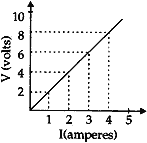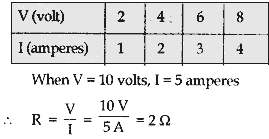#### Question: Find the number of electrons transferred between two points kept at a potential difference of 20 V if 40 J of work is done.

Here, potential difference (V) = 20 V, work (W) = 40 J
We know that
Work (W) = Charge (Q) × Potential difference (V)
or 40 J = Q × 20 V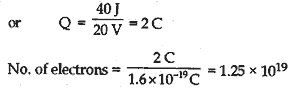#### Question: Name and define SI unit of resistance. Calculate the resistance of a resistor if the current flowing through it is 200 mA, when the applied potential difference is 0.8 V.

1. The SI unit of resistance is Ohm (Ω).
2. If a current of one ampere flows through a wire on applying a potential difference of one volt across it, then the resistance of the wire is said to be 1 Ω.
3. Here, Current (I) = 200 mA = 200/1000 A = 0.2 A
Potential difference (V) = 0.8 V
We know,  V = IR
or  0.8/0.2 = R
or  R = 4 Ω

#### Question: (1). Explain why conductor offers resistance to the flow current. (2). Differentiate between conductor, resistor and resistance.

1. We know that motion of electrons in an electric circuit constitutes an electric current. However, the electrons are not completely free to move within the conductor. They are restrained by the attraction of the atoms among which they move.
2. Conductor: Any substance that is capable of readily transmitting heat, electricity etc. is called a conductor.
Resistor: A conductor having some appreciable resistance is called a resistor.
Resistance: It is the property of a material by virtue of which it opposes the flow of a current through it.

#### Question: A piece of wire of resistance 6 Ω is connected to a battery of 12 V. Find the amount of current flowing through it. Now, the same wire is redrawn by stretching it to double its length. Find the resistance of the new (redrawn) wire.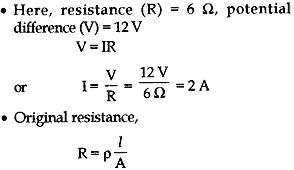If the wire is doubled, then its new length l‘ = 2l and new area of cross-section A’ = A/2.
Then new resistance,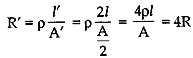or R’ = 4R, i.e., the resistance increases to four time.

#### Question: A wire of length L and resistance R is stretched so that the length is doubled and area of cross-section is halved. How will its: (a) resistance change? (b) resistivity change?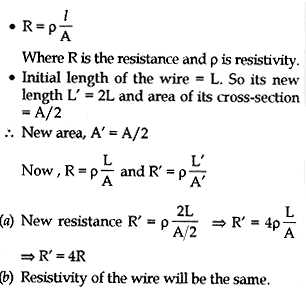#### Question: A nichrome wire has a resistance of 10 Ω. Find the resistance of another nichrome wire, whose length is three times and area of cross-section four times the first wire.

Answer: Resistance (R) of first wire = ρl/A

Resistance (R’) of second wire = ρ×3l/4A = 3ρl/4A

So the resistance of the second wire is three-fourth the resistance of the first wire i.e., 7.5 Ω.

## Sources of Energy: 10th Science Chapter 14

Class: 10th Class Subject: Science Chapter: Chapter 14: Sources of Energy Quiz: – Questions MCQs: …